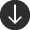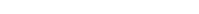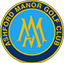#### Measuring Bunkers For Blinder Bunker LinerMeasure each distance width B to B, C to C, and D to D through the bunkers contours. Then measure A to A which equals the bunker’s length.  Multiple each width measurement by the bunker length measurement (A). Add to together the total of the three individual calculations and divide by 3 for the bunker area. Add an extra 10% to the total area for contingency. For bunkers with more complex shaping, additional width measurements may be required. Simply divide the number of width measurements by the overall length measurement of the bunker.

#### Measuring Circular Bunkers For Blinder Bunker LinerFor circular bunkers only one measurement is required A to A which gives you the diameter of the bunker. To calculate the area of the circular bunker you need the radius which is half of the diameter. The formula for calculating the area is r2 = 3.14. Therefore mulitple the radius by the radius then mulitple by 3.14. e.g diameter 6m, raidus 3m – 3 x 3 x 3.14 = area 28.26. . Add an extra 10% to the total area for contingency.

#### Measuring More Complex Bunkers for Blinder Bunker LinerMeasure each distance width B to B, C to C, D to D and E to E through the bunkers contours. Then measure A to A which equals the bunker’s length.  Multiple each width measurement by the bunker length measurement (A). Add to together the total of the four individual calculations and divide by 4 for the bunker area. Add an extra 10% to the total area for contingency.#### Keen to know more about Blinder?

Call us on: +44 (0) 1747 820495 OR Email: [email protected]